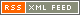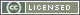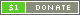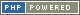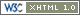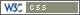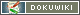# Assignment Operators

Assignment Operators set the value of the Variable on the left of the Operator to be the value of the expression on the right of the Operator, after any appropriate operation (as determined by the Assignment Operator in question).

Symbol Type Name Description Example
General
= Infix Assignment Sets the left term to be equal to the right term ` a = b `
Arithmetic
Number
+= Infix Addition assignment Numeric addition and set equal to ` a += b `
-= Infix Subtraction assignment Numeric subtraction and set equal to ` a -= b `
*= Infix Multiplication assignment Numeric multiplication and set equal to ` a *= b `
/= Infix Division assignment Numeric division and set equal to ` a /= b `
%= Infix Modulus assignment Numeric modulus (remainder) and set equal to ` a %= b `
**= Infix Exponentiation assignment Exponentiation (raise to power) and set equal to ` a **= b `
String
~= Infix Concatenation assignment String concatenation and set equal to ` a ~= b `
~*= Infix Repetition assignment String repetition and set equal to ` a ~*= b `
Array
@= Infix Merge assignment Array merge and set equal to ` a @= b `
@*= Infix Repetition assignment Array repetition and set equal to ` a @*= b `
Bitwise
Number
+&= Infix AND assignment Numeric bitwise AND and set equal to ` a +&= b `
+|= Infix OR assignment Numeric bitwise OR and set equal to ` a +|= b `
+^= Infix XOR assignment Numeric bitwise XOR and set equal to ` a +^= b `
+<= Infix Shift left assignment Numeric bitwise shift left and set equal to ` a +<= b `
+>= Infix Shift right assignment Numeric bitwise shift right and set equal to ` a +>= b `
String
~&= Infix AND assignment String bitwise AND and set equal to ` a ~&= b `
~|= Infix OR assignment String bitwise OR and set equal to ` a ~|= b `
~^= Infix XOR assignment String bitwise XOR and set equal to ` a ~^= b `
~<= Infix Shift left assignment String bitwise shift left and set equal to ` a ~<= b `
~>= Infix Shift right assignment String bitwise shift right and set equal to ` a ~>= b `
Boolean
?&= Infix AND assignment Boolean bitwise AND and set equal to ` a ?&= b `
?|= Infix OR assignment Boolean bitwise OR and set equal to ` a ?|= b `
?^= Infix XOR assignment Boolean bitwise XOR and set equal to ` a ?^= b `
Logical
High precedence (tight)
&&= Infix AND assignment Logical AND and set equal to ` a &&= b `
||= Infix OR assignment Logical OR and set equal to ` a ||= b `
^^= Infix XOR assignment Logical XOR and set equal to ` a ^^= b `
|?|= Infix Existence assignment Logical ERR and set equal to ` a |?|= b `
Range
...= Infix Range assignment Range and set equal to ` a ...= b `